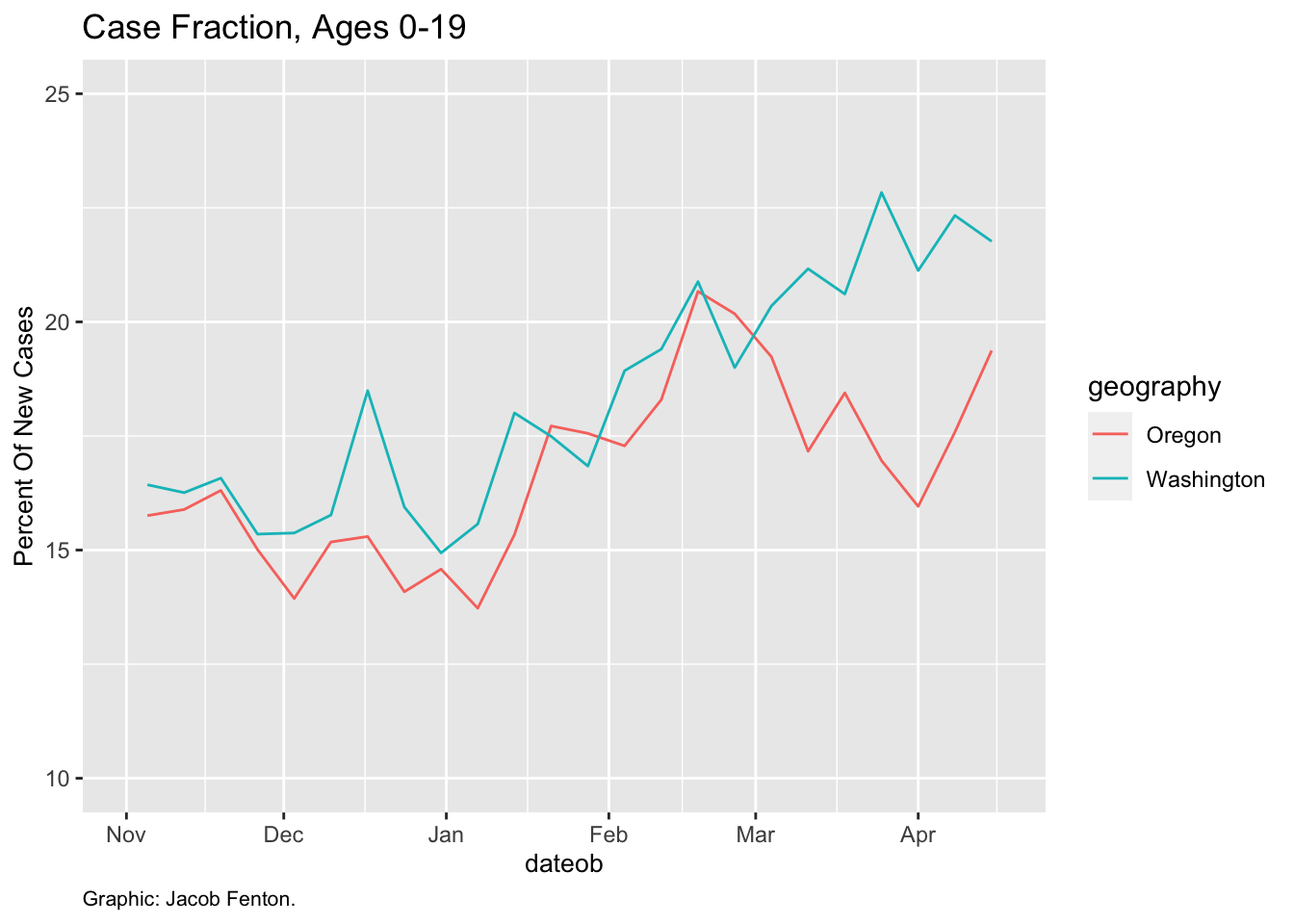## Introduction

This is a look at AAP’s COVID-19 weekly case data through their weekly reports. This is as of their report covering April 15.

The pdf reports that AAP releases use on cumulative case numbers covering the entire pandemic, whereas we are interested in the instantanaeous values.

``````# Libraries

library(ggplot2)
library(scales)
library(dplyr)

file = "aap_data_national.csv"
aap_data\$dateob = as.Date(aap_data\$date)

# remove spaces for consistency
aap_data\$age_range = trimws(aap_data\$age_range)
aap_data\$geography = trimws(aap_data\$geography)``````

### Oregon and Washington

Both Oregon and Washington track data for those aged 0-19.

``````pnw <- aap_data %>% filter(geography == 'Oregon' | geography == 'Washington' )

ggplot(data= pnw, aes(x=dateob,y=new_child_case_prcnt)) + ylab('Percent Of New Cases')+geom_line(aes(color = geography)) + ylim(10,25) + xlim(as.Date('2020-11-01'), as.Date('2021-04-17')) + labs(title = "Case Fraction, Ages 0-19") + labs(caption="Graphic: Jacob Fenton.") + theme( plot.caption = element_text(size = 8,hjust = 0), axis.title=element_text(size=10))``````### National average

This includes jurisdictions that track juveniles as 0-17, 0-18 and 0-19. States AAP identified as having inconsistent data are removed, as well as overseas U.S. territories. A total of 41 states are included. This also ignores a few jurisdictions that track juveniles as 0-14 or 0-20.

``national <- aap_data %>% filter (!geography %in% c("Alabama", "Texas", "Rhode Island", "Massachusetts", "Hawaii", "Missouri", "New York", "Guam", "Puerto Rico")) %>% filter( age_range=='0-17' | age_range=='0-19'| age_range=='0-18') %>%  group_by(dateob ) %>% summarise(children = sum(new_child_cases), total = sum(new_total_cases)) ``
``## `summarise()` ungrouping output (override with `.groups` argument)``
``````national\$child_age_prcnt = 100*national\$children / national\$total

ggplot(data= national, aes(x=dateob,y=child_age_prcnt)) + ylab('Percent Of New Cases')+ geom_smooth() + geom_line() + ylim(5,25) + labs(title = "National Percent Of Juvenile COVID-19 Cases") + labs(caption="Source: AAP. Shows states tracking children aged 0-17, 0-18, 0-19. States with inconsistent data removed. Graphic: Jacob Fenton.") + theme( plot.caption = element_text(size = 8,hjust = 0), axis.title=element_text(size=10)) + ylab("Percent of total cases") + xlab("Date") ``````
``## `geom_smooth()` using method = 'loess' and formula 'y ~ x'``
``## Warning: Removed 4 rows containing non-finite values (stat_smooth).``
``## Warning: Removed 4 row(s) containing missing values (geom_path).``